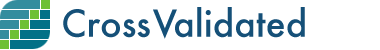1

(click on this box to dismiss)

Top 'n' users (within first 'n' days since account creation) per reputation earned with answers (+ upvotes, + accepts, + bounties, - downvotes). One objective of this query is to monitor potential new contributors and interact with them (for example, motivate them to continue participating). Another objective is to learn about the overall rank reputation based on answers (just set the second parameter in which 'number of days' is equal to the site's age).Q&A for people interested in statistics, machine learning, data analysis, data mining, and data visualization

DECLARE @TOP INT = ##TOP?10##;
-- TOP: TOP 'n' rank:

(
SELECT p.*,
ROW_NUMBER() OVER (PARTITION BY p.OwnerUserId ORDER BY p.Score DESC) AS [top_answer]
FROM Posts p
WHERE p.PostTypeId = 2 -- answers
AND p.CommunityOwnedDate IS NULL
AND p.OwnerUserId IS NOT NULL -- active accounts
)
-- credits to user 'rene'; code adapted from
SELECT TOP (@TOP)
sum(CASE v.VoteTypeId
WHEN 1 THEN 15 -- accepted
WHEN 2 THEN 10 -- up vote
WHEN 3 THEN -2 -- down vote
WHEN 9 THEN v.BountyAmount -- bounty earned
ELSE 0
END
sum(CASE v.VoteTypeId
WHEN 1 THEN 15
WHEN 2 THEN 10
WHEN 3 THEN -2
WHEN 9 THEN v.BountyAmount
ELSE 0
END
ROUND(CAST(sum(CASE
WHEN v.VoteTypeId = 1 AND p.top_answer = 1 THEN 15
WHEN v.VoteTypeId = 2 AND p.top_answer = 1 THEN 10
WHEN v.VoteTypeId = 3 AND p.top_answer = 1 THEN -2
WHEN v.VoteTypeId = 9 AND p.top_answer = 1 THEN v.BountyAmount
ELSE 0
END
) AS float) /
CAST(sum(CASE v.VoteTypeId
WHEN 1 THEN 15
WHEN 2 THEN 10
WHEN 3 THEN -2
WHEN 9 THEN v.BountyAmount
ELSE 0
END
) AS float) * 100, 1) AS [Pct top answer], -- percentage of reputation from top answer (in relation to total answer rep).
ROUND(CAST(sum(CASE
WHEN v.VoteTypeId = 9 THEN v.BountyAmount
ELSE 0
END
) AS float) /
CAST(sum(CASE v.VoteTypeId
WHEN 1 THEN 15
WHEN 2 THEN 10
WHEN 3 THEN -2
WHEN 9 THEN v.BountyAmount
ELSE 0
END
) AS float) * 100, 1) AS [Pct bounties] -- percentage of reputation from bounties (in relation to total answer rep).
LEFT JOIN Votes v on v.PostId = p.Id
WHERE (v.VoteTypeId IN (1,2,3,9)
OR v.VoteTypeId IS NULL) -- so answers yet not voted can be counted on.
AND u.CreationDate > GETDATE() - ##days:int?30## -- new users (first 'n' days in SE).
-- days: First 'n' days from 'new users' in Stack Exchange:
GROUP BY u.Id
AND sum(CASE v.VoteTypeId
WHEN 1 THEN 15
WHEN 2 THEN 10
WHEN 3 THEN -2
WHEN 9 THEN v.BountyAmount
ELSE 0
END
) > 10 -- this is to avoid division by zero in 'Pct' columnsHold tight while we fetch your results